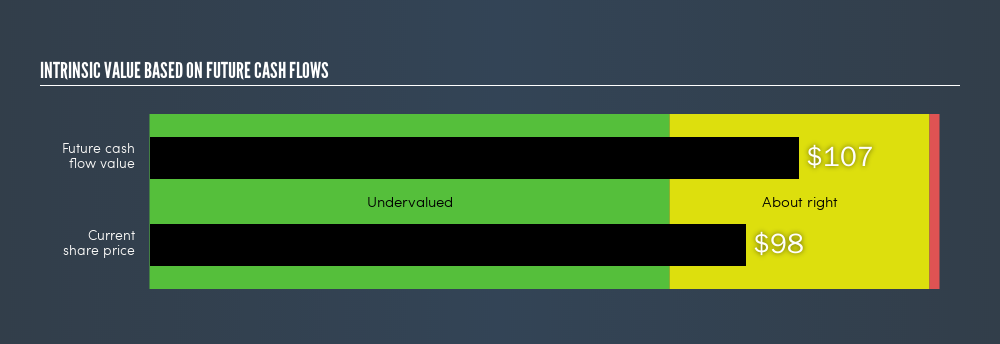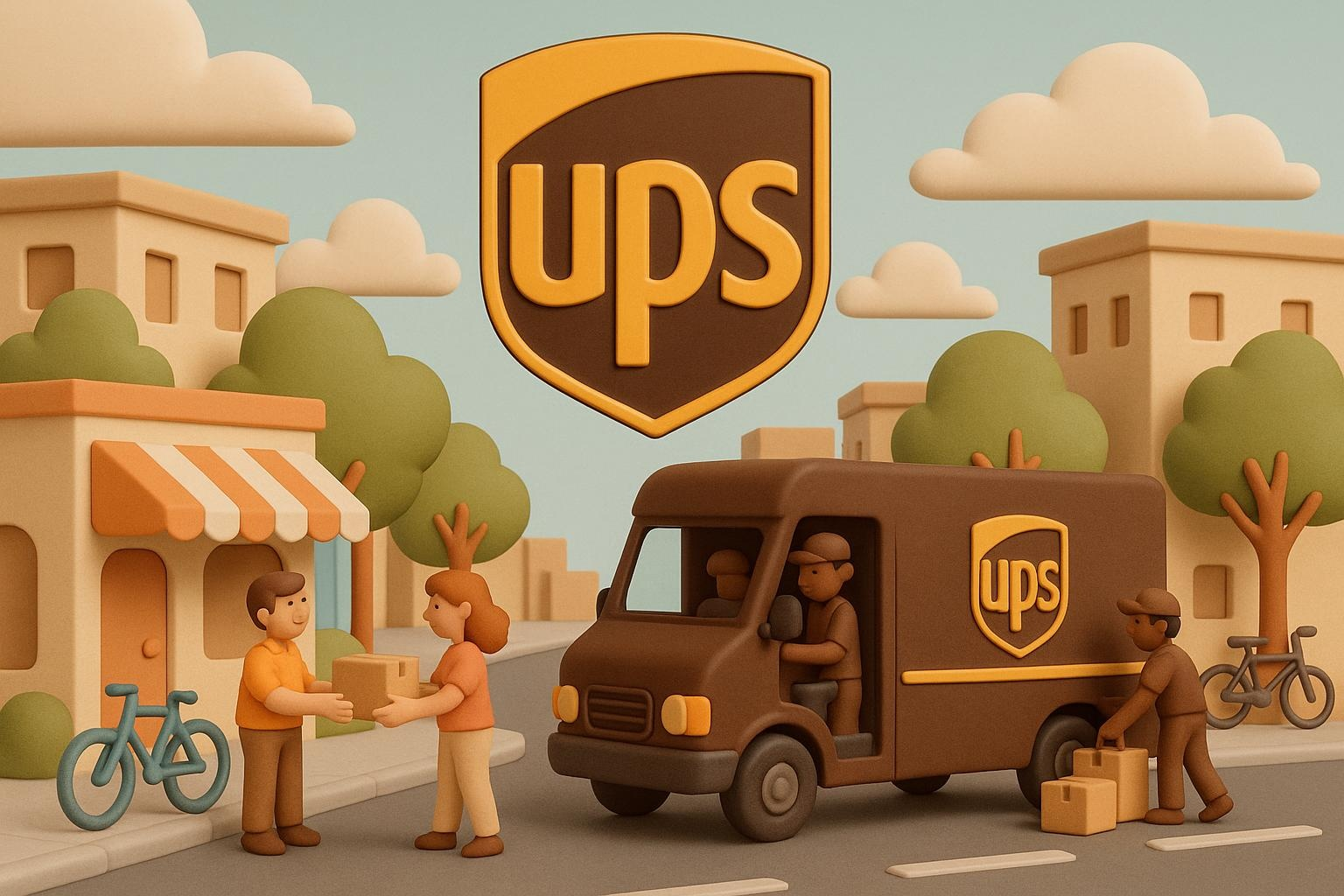latest

# Estimating The Intrinsic Value Of United Parcel Service, Inc. (NYSE:UPS)

Want to participate in a short research study? Help shape the future of investing tools and you could win a \$250 gift card!

Today we’ll do a simple run through of a valuation method used to estimate the attractiveness of United Parcel Service, Inc. (NYSE:UPS) as an investment opportunity by taking the expected future cash flows and discounting them to their present value. I will use the Discounted Cash Flow (DCF) model. It may sound complicated, but actually it is quite simple!

We generally believe that a company’s value is the present value of all of the cash it will generate in the future. However, a DCF is just one valuation metric among many, and it is not without flaws. If you want to learn more about discounted cash flow, the rationale behind this calculation can be read in detail in the Simply Wall St analysis model.

### The model

We’re using the 2-stage growth model, which simply means we take in account two stages of company’s growth. In the initial period the company may have a higher growth rate and the second stage is usually assumed to have a stable growth rate. To start off with, we need to estimate the next ten years of cash flows. Where possible we use analyst estimates, but when these aren’t available we extrapolate the previous free cash flow (FCF) from the last estimate or reported value. We assume companies with shrinking free cash flow will slow their rate of shrinkage, and that companies with growing free cash flow will see their growth rate slow, over this period. We do this to reflect that growth tends to slow more in the early years than it does in later years.

Generally we assume that a dollar today is more valuable than a dollar in the future, and so the sum of these future cash flows is then discounted to today’s value:

#### 10-year free cash flow (FCF) forecast

 2019 2020 2021 2022 2023 2024 2025 2026 2027 2028 Levered FCF (\$, Millions) \$4.02k \$4.25k \$5.55k \$5.82k \$6.47k \$7.00k \$7.46k \$7.87k \$8.23k \$8.57k Growth Rate Estimate Source Analyst x9 Analyst x8 Analyst x3 Analyst x1 Analyst x1 Est @ 8.26% Est @ 6.6% Est @ 5.44% Est @ 4.63% Est @ 4.06% Present Value (\$, Millions) Discounted @ 9.42% \$3.67k \$3.55k \$4.23k \$4.06k \$4.12k \$4.08k \$3.97k \$3.83k \$3.66k \$3.48k

Present Value of 10-year Cash Flow (PVCF)= \$38.66b

“Est” = FCF growth rate estimated by Simply Wall St

We now need to calculate the Terminal Value, which accounts for all the future cash flows after this ten year period. The Gordon Growth formula is used to calculate Terminal Value at a future annual growth rate equal to the 10-year government bond rate of 2.7%. We discount the terminal cash flows to today’s value at a cost of equity of 9.4%.

Terminal Value (TV) = FCF2029 × (1 + g) ÷ (r – g) = US\$8.6b × (1 + 2.7%) ÷ (9.4% – 2.7%) = US\$131b

Present Value of Terminal Value (PVTV) = TV / (1 + r)10 = \$US\$131b ÷ ( 1 + 9.4%)10 = \$53.41b

The total value is the sum of cash flows for the next ten years plus the discounted terminal value, which results in the Total Equity Value, which in this case is \$92.06b. The last step is to then divide the equity value by the number of shares outstanding. This results in an intrinsic value estimate of \$106.96. Relative to the current share price of \$98.21, the company appears about fair value at a 8.2% discount to where the stock price trades currently. The assumptions in any calculation have a big impact on the valuation, so it is better to view this as a rough estimate, not precise down to the last cent.NYSE:UPS Intrinsic value, June 7th 2019

### The assumptions

The calculation above is very dependent on two assumptions. The first is the discount rate and the other is the cash flows. If you don’t agree with these result, have a go at the calculation yourself and play with the assumptions. The DCF also does not consider the possible cyclicality of an industry, or a company’s future capital requirements, so it does not give a full picture of a company’s potential performance. Given that we are looking at United Parcel Service as potential shareholders, the cost of equity is used as the discount rate, rather than the cost of capital (or weighted average cost of capital, WACC) which accounts for debt. In this calculation we’ve used 9.4%, which is based on a levered beta of 1.123. Beta is a measure of a stock’s volatility, compared to the market as a whole. We get our beta from the industry average beta of globally comparable companies, with an imposed limit between 0.8 and 2.0, which is a reasonable range for a stable business.

### Next Steps:

Whilst important, DCF calculation shouldn’t be the only metric you look at when researching a company. The DCF model is not a perfect stock valuation tool. Rather it should be seen as a guide to “what assumptions need to be true for this stock to be under/overvalued?” If a company grows at a different rate, or if its cost of equity or risk free rate changes sharply, the output can look very different. For United Parcel Service, There are three relevant factors you should look at:

1. Financial Health: Does UPS have a healthy balance sheet? Take a look at our free balance sheet analysis with six simple checks on key factors like leverage and risk.
2. Future Earnings: How does UPS’s growth rate compare to its peers and the wider market? Dig deeper into the analyst consensus number for the upcoming years by interacting with our free analyst growth expectation chart.
3. Other High Quality Alternatives: Are there other high quality stocks you could be holding instead of UPS? Explore our interactive list of high quality stocks to get an idea of what else is out there you may be missing!

PS. Simply Wall St updates its DCF calculation for every US stock every day, so if you want to find the intrinsic value of any other stock just search here.

We aim to bring you long-term focused research analysis driven by fundamental data. Note that our analysis may not factor in the latest price-sensitive company announcements or qualitative material.

If you spot an error that warrants correction, please contact the editor at editorial-team@simplywallst.com. This article by Simply Wall St is general in nature. It does not constitute a recommendation to buy or sell any stock, and does not take account of your objectives, or your financial situation. Simply Wall St has no position in the stocks mentioned. Thank you for reading.